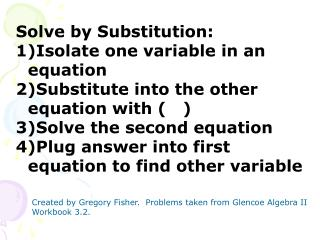DownloadDownload PresentationSolve by Substitution: Isolate one variable in an equation

# Solve by Substitution: Isolate one variable in an equation

Télécharger la présentation## Solve by Substitution: Isolate one variable in an equation

- - - - - - - - - - - - - - - - - - - - - - - - - - - E N D - - - - - - - - - - - - - - - - - - - - - - - - - - -
##### Presentation Transcript

1. Solve by Substitution: • Isolate one variable in an equation • Substitute into the other equation with ( ) • Solve the second equation • Plug answer into first equation to find other variable Created by Gregory Fisher. Problems taken from Glencoe Algebra II Workbook 3.2.

2. X – 3y = 16 • 4x – y = 9 -y = 9 – 4x y = -9 + 4x X – 3(-9 + 4x) = 16 X + 27 – 12x = 16 -11x = -11 x = 1 1) Isolate one variable in an equation 2) Substitute into the other equation with ( ) 3) Solve the second equation 4) Plug answer into first equation to find other variable Y=-9+4(1) Y=-5 (1,-5) Consistent Independent

3. 5) 2m + n = 6 5m + 6n = 1 n = 6 – 2m 5m + 6(6 – 2m) = 1 5m + 36 – 12m = 1 -7m = -35 m = 5 n= 6 – 2(5) n= -4 (5,-4) Consistent Independent

4. 7) u – 2v = 1/2 -u + 2v = 5 u = 1/2 + 2v -(1/2 + 2v) + 2v= 5 -1/2 -2v + 2v= 5 0v = 5.5 0 = 5.5 No Solution! Inconsistent

5. Solve by Elimination • Multiply one or both equations so that one of the variables is the additive inverse of the other one • Add the two equations together • Solve the equation • Substitute answer into an equation and solve

6. 11) 2m – n = -1 3m + 2n = 30 Multiply top by 2 4m – 2n = -2 3m + 2n = 30 Solve by Elimination 1) Multiply one or both equations so that one of the variables is the additive inverse of the other one 2) Add the two equations together 3) Solve the equation 4) Substitute answer into an equation and solve 7m = 28 m=4 2(4) – n = -1 8 – n = -1 –n = -9 n = 9

7. 25) 5g + 4k = 10 -3g – 5k = 7 Multiply top by 5 Multiply bottom by 4 25g + 20k =50 -12g- 20k =28 Solve by Elimination 1) Multiply one or both equations so that one of the variables is the additive inverse of the other one 2) Add the two equations together 3) Solve the equation 4) Substitute answer into an equation and solve 13g = 78 g=6 5(6) + 4k = 10 30+ 4k = 10 4k = -20 k = -5 (6,-5)

8. 14) 2x - y = -4 -4x +2y =6 Multiply top by 2 4x – 2y =-8 -4x+2y =12 Solve by Elimination 1) Multiply one or both equations so that one of the variables is the additive inverse of the other one 2) Add the two equations together 3) Solve the equation 4) Substitute answer into an equation and solve 0x = 4 0=4 Inconsistent

9. When is it best to use each method…. Substitution: When it’s easy to isolate one variable Elimination: When it’s not easy to isolate one variable Or you just want the value of one variable (Eliminate the other one)

10. Check for Understanding • What must you multiply the top equation by to eliminate the x? • 5x – 3y = 12 • 15x – 6y = 2 By -3 to make -15 2) What will y be equal to in the second equation to solve by substitution? 5x – 3y = 12 15x – y = 2 2) -y=2 - 15x Y = -2 + 15x